# ISEE Upper Level Math : Circles

## Example Questions

1 2 3 4 5 6 7 8 10 Next →

### Example Question #5 : How To Find The Angle Of A Sector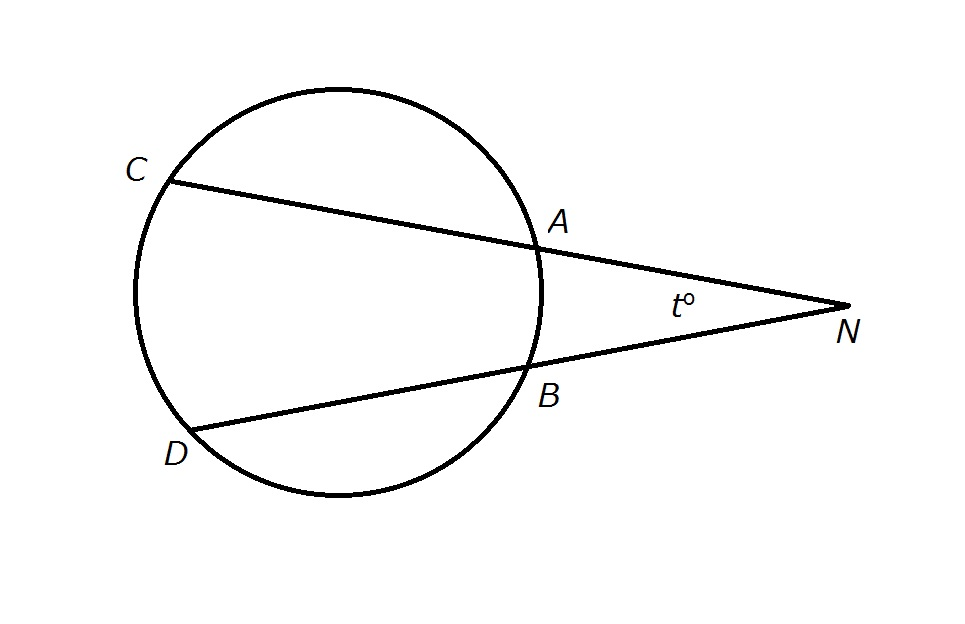Figure NOT drawn to scale.

The circumference of the above circle is 120.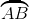and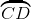have lengths 10 and 20, respectively. Evaluate.Explanation:

The length ofcomprises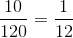of the circumference of the circle. Therefore, its degree measure is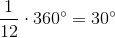. Similarly, The length ofcomprises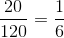of the circumference of the circle. Therefore, its degree measure is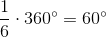If two secants are constructed to a circle from an outside point, the degree measure of the angle the secants form is half the difference of those of the arcs intercepted - that is,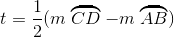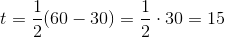.

### Example Question #6 : How To Find The Angle Of A Sector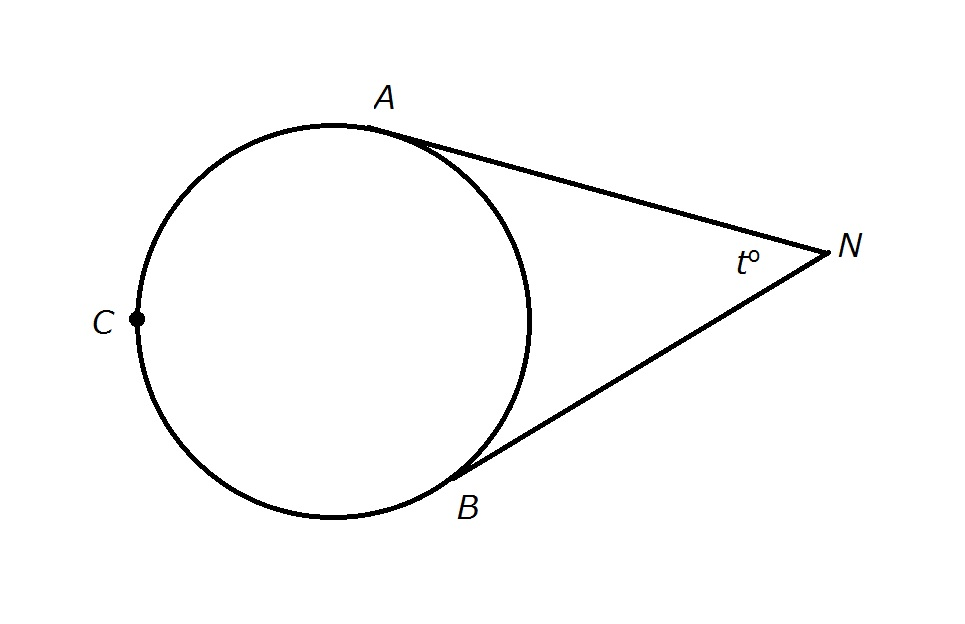Figure NOT drawn to scale.

Refer to the above diagram.and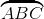have lengths 80 and 160, respectively. Evaluate.Explanation:

The circumference of the circle is the sum of the two arc lengths: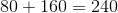The length ofcomprises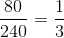of the circumference of the circle. Therefore, its degree measure is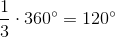. Consequently,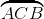is an arc of degree measure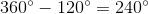The segments shown are both tangents fromto the circle. Consequently, the degree measure of the angle they form is half the difference of the angle measures of the arcs they intercept - that is,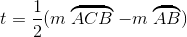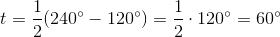### Example Question #7 : How To Find The Angle Of A Sector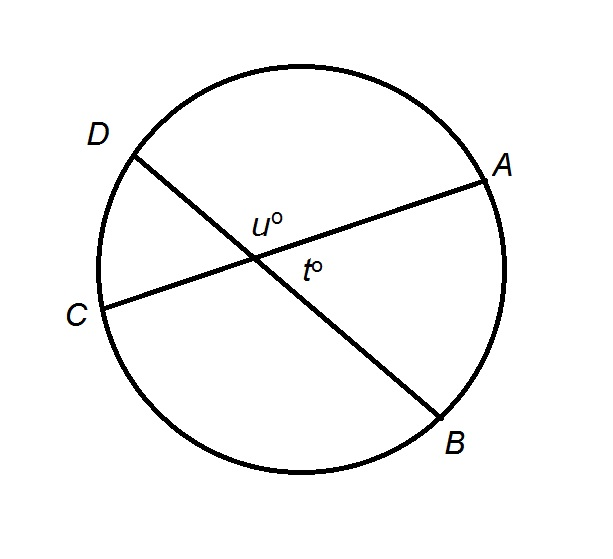Figure NOT drawn to scale.

The circumference of the above circle is 100.andhave lengths 20 and 15, respectively. Evaluate.Explanation:

The length ofcomprises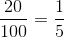of the circumference of the circle. Therefore, its degree measure is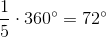. Similarly, The length ofcomprises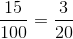of the circumference of the circle. Therefore, its degree measure is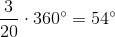If two chords cut each other inside the circle, as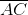and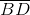do, and one pair of vertical angles are examined, then the degree measure of each angle is half the sum of those of the arcs intercepted - that is,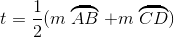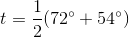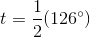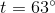### Example Question #1 : How To Find The Percentage Of A Sector From An Angle

Sector SOW has a central angle of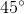. What percentage of the circle does it cover?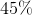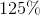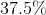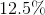Explanation:

Sector SOW has a central angle of. What percentage of the circle does it cover?

Recall that there is a total of 360 degrees in a circle. SOW occupies 45 of them. To find the percentage, simply do the following: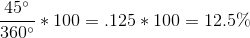### Example Question #1 : How To Find The Percentage Of A Sector From An Angle

While visiting a history museum, you see a radar display which consists of a circular screen with a highlighted wedge with an angle of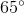. What percentage of the circle is highlighted?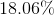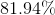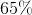Explanation:

While visiting a history museum, you see a radar display which consists of a circular screen with a highlighted wedge with an angle of. What percentage of the circle is highlighted?

To find the percentage of a sector, simply put the degree measure of the angle over 360 and multiply by 100.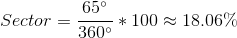So, our answer is 18.06%

1 2 3 4 5 6 7 8 10 Next →

### All ISEE Upper Level Math Resources